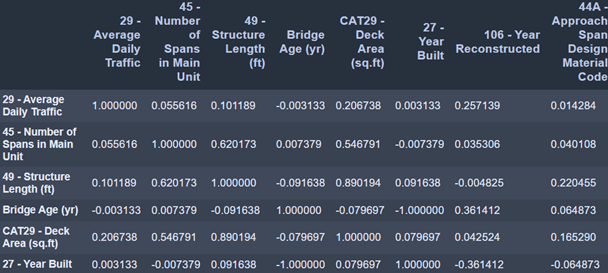Search

# Predict Your Bridge Defects with Machine Learning

Updated: Oct 19, 2020

Bridge inspectors collect large amounts of data to ultimately create an inspection report. Beyond reporting, what other insights can be extracted?

The most common data extracted in-field is defect data namely defect name, type, location, description, and picture. In a previous blog we discussed how this data can be used to prioritize repairs, identify where most defects occur and track structure health.

In this blog we will review how this data can be used even further to predict defects before they occur. The steps to achieve this are:

1. Identify what Causes Defects on your Structures

2. Apply the Linear Regression Machine Learning Technique

Before moving forward, we need to remind you that to do any sort of analysis on your data it needs to be digitized.

# Identify what Causes Defects on your Structures

The first step to predicting defects is to understand what the different parameters are that affect them. Is it a specific weather/season? A structure location? Traffic load?

For instance, have you ever wondered if the corrosions were detected during specific weather seasons?

To avoid the guessing work, we need to identify the relationship between a defect and different parameters such as bridge location, weather, date, etc. In the data science field this is called correlation.

Example:Data Source: https://infobridge.fhwa.dot.gov/Data

In this table, the column and row headers are equivalent. These are the parameters that we are evaluating against each other in attempt to find a correlation. The numbers indicate the correlation percentage and can only be between -1 and 1. For instance 0.055 is 5.5% correlation and in that same logic 0 is 0% and 1 is 100%. The negative number simply mean that the correlation between the 2 parameters is inversely proportional.

To avoid going into all the numbers looking for -1’s and 1’s, where correlation is high, we can plot the table above and end up with the following:In the plot above, high correlation are areas where the yellow is dominant. You can see where we drew a red circle around the largest yellow area.

Going back to our matrix, this area represents the following portion of our table and indicates a strong correlation between the parameters: Length of Structure Improvement with the Bridge Improvement Cost (which makes perfect sense).Data Source: https://infobridge.fhwa.dot.gov/Data

This process, table and plot can be done using different parameters such as defect type, temperature, date, etc.

# Apply the Linear Regression Machine Learning Technique

After identifying the parameters that have strong correlations, we have to plot them on a simple x-y axis. In the example below the 2 parameters are Corrosion Intensity (y-axis) and Humidity (x-axis).Then, we must identify the mathematical equation that covers most of the data points on the plot. In the example above we used the Linear Regression technique that involves drawing the straight line that covers most of the data points. In today’s world you don’t have to do this process manually you can use programming languages like python that will automatically plot the Linear Regression for you.

Referring to the plot above, if the Humidity level is at 70, I can predict a Corrosion intensity of approx. 6.2. Please keep in mind that this will not be 100% accurate. Accuracy is determined by how close the line you drew is to your data points.

The benefit of predicting your defects are numerous:

• Optimize time in the field by focusing on the areas where you predict have a higher chance of defects.

• Optimize repair budgets.

Defect prediction is only one insight that you can achieve using your data and machine learning. The opportunities here are endless.

# Unlock these insights and many more with Data ReconStart digitizing your data today using Data Recon, a bridge inspection software for electronic data collection, automatic reporting generation and data analytics. Learn more about it here.

43 views